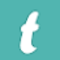## Two ships leave a port at the same time. One goes 24km/hr in the direction N45°E and the other travels 32km/hr in the direction S75°E. Find the distance between the ships at the end of 3 hours.### Text version of the answer is

Let Ox be the position of the first ship and dy be the position  of the second ship. At the end of 3 hours.

Speed of ship 1a : 34 km/h

Distance travelled in

1 hour : 24 km

Distance travelled in

3 hours  = 0X = 24 × 3

OX = 72 km

Speed of ship  : 32 km/hr

Distance traelled in 1  hour : 32 km

Distance travelled in 3 hours = 32 × 3  = 96 km

In ∆ OXY, by the using cosine formula,

(xy) 2 = (ox) 2 + (Oy) 2 − 2 (OX) (OY) cos ∠XOY ….. (1)

We know ∠NOS = 180°

∠XON + ∠XOY + ∠YOS  = 180°

45° + ∠XOY + 75°  = 180°

120° + ∠xoy = 180°

∠xoy = 60°

Hence, Put OX = 72 km, OY = 96 km and

∠XOY = 60°  in equation (1)

(Xy 2 ) = (72) 2 + (96) 2 − 2 (72) (96) cos 60°

(Xy 2 ) = (72) 2 + (96) 2 − 2 (72) (96) (1/2)

(Xy 2 ) = 5154 + 9216 − 6912

(Xy 2 ) = 7488

Xy = √7488

= √(26×32×13)

= √((23×3)2)  √(×13)

= 24√13 km

= 24 × 3.60 km

= 86.4 km

Rough

2 | 7488

2 | 3744

2 | 1872

2 | 936

2 | 468

2 | 234

3 | 117

3 | 39

13 | 13

2 | 1

= √7488 = 2 × 2 × 2 × 3 × √13